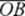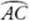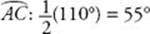﻿ ﻿CIRCUMFERENCE AND AREA - Circles - PLANE GEOMETRY - SAT SUBJECT TEST MATH LEVEL 1

## PLANE GEOMETRY## CHAPTER 11Circles• Circumference and Area

• Tangents to a Circle

• Exercises

A circle consists of all the points that are the same distance from one fixed point called the center. That distance is called the radius of the circle. The figure below is a circle of radius 1 unit whose center is at the point O. A, B, C, D, and E, which are each 1 unit from O, are all points on circle O. The word radius is also used to represent any of the line segments joining the center and a point on the circle. The plural of radius is radii. In circle O below,, andare all radii. If a circle has radius r, each of the radii is r units long. A point is inside a circle if the distance from the center to that point is less than the radius. A point is outside a circle if the distance from the center to that point is greater than the radius.EXAMPLE 1: In the figure below, O is the center of the circle. To find m∠B and m∠O, observe that sinceandare radii, OA = OB and ΔAOB is isosceles. So m∠B = 25° and m∠O = 180° – (25° + 25°) = 130°.Key Fact J1

Any triangle formed by connecting the endpoints of two radii is isosceles.

A chord of a circle is a line segment that has both endpoints on the circle. In the figure at the beginning of this chapter,andare chords. A chord such asthat passes through the center is called a diameter. Since BE = EO + OB, a diameter is twice as long as a radius.

Key Fact J2

If d is the diameter and r is the radius of a circle: d = 2r.

Key Fact J3

Diameters are the longest line segments that can be drawn that have both endpoints on or inside a circle.

### CIRCUMFERENCE AND AREA

The total length around a circle is called the circumference. In every circle, the ratio of the circumference to the diameter is exactly the same and is denoted by the symbol π (the Greek letter “pi”).

Key Fact J4So there are two formulas for the circumference of a circle:

C = πd and C = 2πr

Key Fact J5

The value of π is approximately 3.14.

Smart Strategy

On almost all questions on the Math 1 test that involve circles, you are expected to leave your answer in terms of π. If you need an approximation to test the values of the choices use your calculator. To avoid rounding errors, use the π key, not 3.14.

EXAMPLE 2: If the circumference of a circle is equal to the perimeter of a square whose sides are 12, what is the radius of the circle?

Since the perimeter of the square is 412 = 48:An arc consists of two points on a circle and all the points between them. If two points, such as A and B in circle O, are the endpoints of a diameter, they divide the circle into two arcs called semicircles. On the Math 1 test, arcalways refers to the smaller arc joining X and Y. In the figure below if we wanted to refer to the larger arc going from X to Y, the one through A and B, we would refer to it as arcor arc.Key Fact J6

The degree measure of a circle is 360°.An angle such asAOB in the figure below, whose vertex is at the center of a circle, is called a central angle.Key Fact J7

The degree measure of an arc equals the degree measure of the central angle that intercepts it.

Remember

Degree measure is not a measure of length. In the circles at the left, arcand arceach measure 60° even though arcis much longer.In the figure above, how long is arc? Since the radius of circle P is 12, its diameter is 24 and its circumference is 24π. Since there are 360° in a circle, arcis, or, of the circumference:.

Key Fact J8

The formula for the area of a circle of radius r is A = πr2.

The area of circle P, on Key Fact J7, is π(12)2 = 144π square units. A sector is a region of a circle bounded by two radii and an arc. In that circle, since the measure ofCPD is 60° (of the circle), the area of sector CPD isthe area of the circle:.

Key Fact J9

In a circle of radius r, if an arc measures x°:

The length of the arc isThe area of the sector formed by the arc and two radii is.

EXAMPLE 3: What are the perimeter and area of the shaded region in the figure below?Smart Strategy

On the Math 1 test, the answer choices to questions such as these are almost always given in terms of π and square roots, so you do not need to use your calculator to evaluate them.

The circumference of the circle is 2πr = 2(10)π = 20π. Since arcisof the circle, the length of arc. Sinceis the hypotenuse of isosceles right triangle POQ, by KEY FACT H7, PQ = 10. So the perimeter of the shaded region is 10+ 5π.

Since the area of the circle is πr 2 = π(102) = 100π, the area of sector POQ is. The area of. So the area of the shaded region is 25π – 50.

An angle formed by two chords with a common endpoint is called an inscribed angle. In the figure below,ABC,ADC,BAD, andBCD are all inscribed angles.Key Fact J10

The measure of an inscribed angle is one-half the measure of its intercepted arc.

EXAMPLE 4: To find mABC in circle O in the figure below, observe that since the measure of an arc is equal to the measure of the central angle that intercepts it, the measure of arcis 110°. SinceABC is an inscribed angle, its measure is one-half the measure of arc.﻿